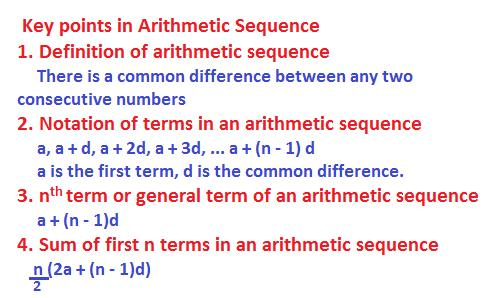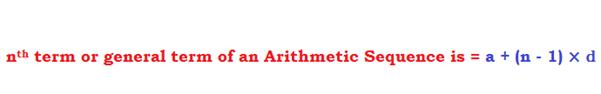# Arithmetic sequenceArithmetic Sequence:

An arithmetic sequence is defined as a group of numbers in which any two consecutive or successive terms have a common difference.

Example:

In the sequence of following numbers:

3, 7, 11, 15, 19, ....

the common difference between any two consecutive numbers is 4.

Therefore, the numbers 3, 7, 11, 15, 19 ...are said to be in Arithmetic Sequence

Denotation of terms in an Arithmetic Sequence

In an Arithmetic Sequence, the following denotations are generally followed:

a denotes the first term,

d, the common difference and

n, the position of any term or a particular term.

Then the n terms of an Arithmetic Sequence are written as
a, a +d, a + 2d, a + 3d, a + 4d, ............., a + (n – 1)×d

In the above sequence of n terms

a is 1st term,
a + d is 2nd term,
a + 2d is 3rd term, and
a + (n – 1) ×d is the last term
a + (n – 1) × d is also called the last term or the nth  term  or still the general term of the above arithmetic sequence.We will discuss below the formulae for finding any particular term and sum of any number of terms in an arithmetic sequence.

What is nth term of a sequence?

The nth term of a sequence denotes the position of a term or a particular term in the sequence.

Any term that is to be found is referred to as nth term or general term.

How to find the nth term/general term of an Arithmetic Sequence?

Let the n terms of an Arithmetic Sequence with a common difference ‘d’ be

a, a + d, a +2d, ........ a + (n – 1) d

Any term in an Arithmetic Sequence is called general term or nth term and it is denoted as an

To find this an the following formula is applied:

an = a + (n – 1) d,  where

a is the first term,

d is the common difference and

n is the position of the term.

Example

1. The first three terms of an Arithmetic Sequence are 3, 7, 11 ...What is the 50th term?

Solution:

In the A.S. 3, 7, 11, the common difference is 4.

To find the 50th term, apply the above formula for nth term
an = a + (n – 1)×d.

In this the first term a = 3, common difference d = 4 and n = 50.

Applying the above formula, the 50th term is
3 + (50 – 1) × 4 = 3 + 196 = 199

2. In an arithmetic sequence with a common difference of 5, the 25th term is 180. What is the 15th term of this sequence?

Solution:

Represent 25th term as a25.

Next, from the nn term formula for an A.S.:  an = a + (n – 1) ×d,
we can write, a25 = 180, n = 25, d = 5.

What needs to be found is the first term, a.

Applying the nth term formula, we get
180 = a + (25 – 1) × 5,

180 = a + 120, so, a = 60.

Again, from the nth term formula, the 15th term is
a15 = 60 + (15 – 1) × 5 = 130

How to find the sum of n terms of an Arithmetic Sequence:

Let the n terms of an Arithmetic Sequence be

a, a + d, a + 2d, ............, a + (n – 1)× d

If Sn is the sum of the above n terms which are in Arithmetic Sequence, then

Sn  = n/2 [2a + (n – 1 ) d]

Now, let ‘l’ denote the last term, then

Since l = a + (n – 1)d, therefore

Sn = n/2[a + l]

Example:

1. The terms of an Arithmetic Sequence are 2, 5, 8, 11, ..........
What is the sum of the first 51 terms of this sequence?

Solution:

Now, first term, a = 2,

Common difference, d = 3,

and the last term is

l = a + (n – 1) × d is

2 + (51 – 1) × 3 = 2 + 150 = 152

Therefore, S = 51/2 [2 + 152] = 3927

Sequences and series.

Arithmetic progression.